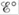# Consider the equation Δ G° = -nF . What are the four terms in this equation? Why does a minus sign appear in the equation? What does the superscript o indicate?### Chemistry: An Atoms First Approach

2nd Edition
Steven S. Zumdahl + 1 other
Publisher: Cengage Learning
ISBN: 9781305079243

#### Solutions

Chapter
Section### Chemistry: An Atoms First Approach

2nd Edition
Steven S. Zumdahl + 1 other
Publisher: Cengage Learning
ISBN: 9781305079243
Chapter 17, Problem 4RQ
Textbook Problem
1 views

## Consider the equation ΔG° = -nF. What are the four terms in this equation? Why does a minus sign appear in the equation? What does the superscript o indicate?

Interpretation Introduction

Interpretation:

The equation for standard free energy change ΔG°=nFE° is given. The significance of all the four terms, minus sign and the superscript ° is to be stated.

Concept introduction:

The free energy change reflects the work involved in moving an electron. The given equation ΔG°=nFE° , shows that the potential of the cell is related to the change in free energy for the reactants and products that are involved in the cell reaction.

To explain: The significance of all the four terms used in the equation ΔG°=nFE° ; the reason of minus sign that appears in the given equation; the significance of superscript ° in the given equation.

### Explanation of Solution

The four terms used in the given equation ΔG°=nFE° are ΔG° , n , F , E° .

The significance of the four terms used in the equation is defined as,

• ΔG° is the free energy available for moving an electron.
• n is the number of electrons involved in a balanced chemical reaction.
• F is the Faraday’s constant (96,485C/mol) .
• E° is the cell potential in the standard state

### Still sussing out bartleby?

Check out a sample textbook solution.

See a sample solution

#### The Solution to Your Study Problems

Bartleby provides explanations to thousands of textbook problems written by our experts, many with advanced degrees!

Get Started

Find more solutions based on key concepts
A person who is setting goals in preparation for a behavior change is in a stage called Precontemplation. T F

Nutrition: Concepts and Controversies - Standalone book (MindTap Course List)

What role do plasmids play in recombinant DNA procedures?

General, Organic, and Biological Chemistry

When water freezes, it expands by about 9.00%. What pressure increase would occur inside your automobile engine...

Physics for Scientists and Engineers, Technology Update (No access codes included)

What is lithification? How is sedimentary rock formed?

Oceanography: An Invitation To Marine Science, Loose-leaf Versin